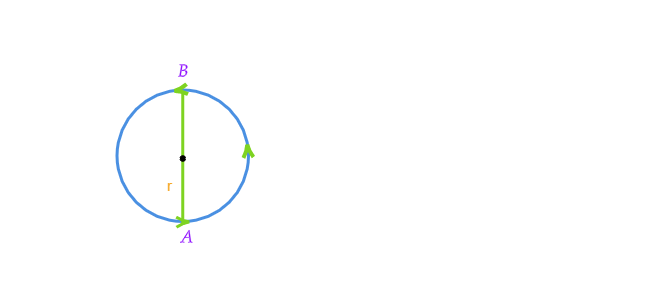# A circular track has a circumference of $3140\ m$ with AB as one of its diameter. A scooter moves from A to B alone the circular path with a uniform speed of $10\ m/s$. Find the distance covered by the scooter.

Here given, circumference of the circular track $=3140\ m$

Distance covered by the scooter is the half of the circumference of the circular track.

Therefor, distance covered by the scooter$=\frac{3140}{2}\ m$

$=1570\ m$Updated on: 10-Oct-2022

62 Views